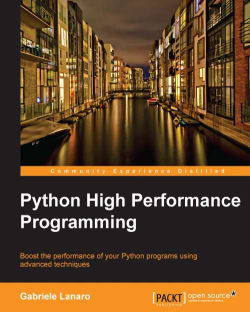### Python High Performance Programming5 (2 reviews total)
By Dr. Gabriele Lanaro
• Constantly updated with 100+ new titles each month
• Breadth and depth in over 1,000+ technologies

Python is a programming language with a vibrant community known for its simplicity, code readability, and expressiveness. The massive selection of third party libraries make it suitable for a wide range of applications. This also allows programmers to express concepts in fewer lines of code than would be possible in similar languages. The availability of high quality numerically-focused tools has made Python an excellent choice for high performance computing. The speed of applications comes down to how well the code is written. Poorly written code means poorly performing applications, which means unsatisfied customers.

This book is an example-oriented guide to the techniques used to dramatically improve the performance of your Python programs. It will teach optimization techniques by using pure python tricks, high performance libraries, and the python-C integration. The book will also include a section on how to write and run parallel code.

This book will teach you how to take any program and make it run much faster. You will learn state-of the art techniques by applying them to practical examples. This book will also guide you through different profiling tools which will help you identify performance issues in your program. You will learn how to speed up your numerical code using NumPy and Cython. The book will also introduce you to parallel programming so you can take advantage of modern multi-core processors.

This is the perfect guide to help you achieve the best possible performance in your Python applications.

Publication date:
December 2013
Publisher
Packt
Pages
108
ISBN
9781783288458

## ChapterÂ 1.Â Benchmarking and Profiling

Recognizing the slow parts of your program is the single most important task when it comes to speeding up your code. In most cases, the bottlenecks account for a very small fraction of the program. By specifically addressing those critical spots you can focus on the parts that need improvement without wasting time in micro-optimizations.

Profiling is the technique that allows us to pinpoint the bottlenecks. A profiler is a program that runs the code and observes how long each function takes to run, detecting the slow parts of the program. Python provides several tools to help us find those bottlenecks and navigate the performance metrics. In this chapter, we will learn how to use the standard `cProfile` module, `line_profiler` and `memory_profiler`. We will also learn how to interpret the profiling results using the program KCachegrind.

You may also want to assess the total execution time of your program and see how it is affected by your changes. We will learn how to write benchmarks and how to accurately time your programs.

When you are designing a performance-intensive program, the very first step is to write your code without having optimization in mind; quoting Donald Knuth:

Premature optimization is the root of all evil.

In the early development stages, the design of the program can change quickly, requiring you to rewrite and reorganize big chunks of code. By testing different prototypes without bothering about optimizations, you learn more about your program, and this will help you make better design decisions.

The mantras that you should remember when optimizing your code, are as follows:

• Make it run: We have to get the software in a working state, and be sure that it produces the correct results. This phase serves to explore the problem that we are trying to solve and to spot major design issues in the early stages.

• Make it right: We want to make sure that the design of the program is solid. Refactoring should be done before attempting any performance optimization. This really helps separate the application into independent and cohesive units that are easier to maintain.

• Make it fast: Once our program is working and has a good design we want to optimize the parts of the program that are not fast enough. We may also want to optimize memory usage if that constitutes an issue.

In this section we will profile a test applicationâ€”a particle simulator. The simulator is a program that takes some particles and evolves them over time according to a set of laws that we will establish. Those particles can either be abstract entities or correspond to physical objects. They can be, for example, billiard balls moving on a table, molecules in gas, stars moving through space, smoke particles, fluids in a chamber, and so on.

Those simulations are useful in fields such as Physics, Chemistry, and Astronomy, and the programs used to simulate physical systems are typically performance-intensive. In order to study realistic systems it's often necessary to simulate the highest possible number of bodies.

In our first example, we will simulate a system containing particles that constantly rotate around a central point at various speeds, like the hands of a clock.

The necessary information to run our simulation will be the starting positions of the particles, the speed, and the rotation direction. From these elements, we have to calculate the position of the particle in the next instant of time.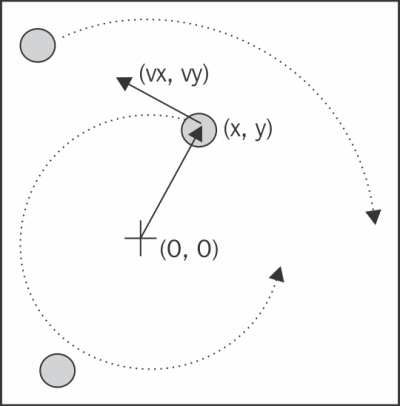The basic feature of a circular motion is that the particles always move perpendicularly to the direction connecting the particle and the center, as shown in the preceding image. To move the particle we simply change the position by taking a series of very small steps in the direction of motion, as shown in the following figure: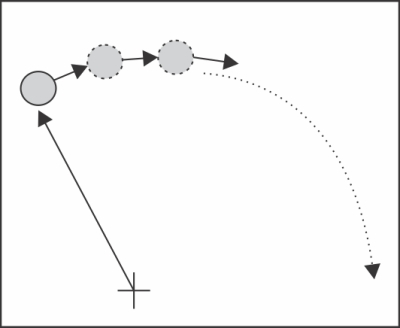We will start by designing the application in an object-oriented way. According to our requirements, it is natural to have a generic `Particle` class that simply stores the particle position (x, y) and its angular speed:

```class Particle:
def __init__(self, x, y, ang_speed):
self.x = x
self.y = y
self.ang_speed = ang_speed```

Another class, called `ParticleSimulator` will encapsulate our laws of motion and will be responsible for changing the positions of the particles over time. The `__init__` method will store a list of `Particle` instances and the `evolve` method will change the particle positions according to our laws.

We want the particles to rotate around the point (x, y), which, here, is equal to (0, 0), at constant speed. The direction of the particles will always be perpendicular to the direction from the center (refer to the first figure of this chapter). To find this vector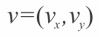(corresponding to the Python variables `v_x` and `v_y`) it is sufficient to use these formulae: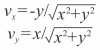If we let one of our particles move, after a certain time dt, it will follow a circular path, reaching another position. To let the particle follow that trajectory we have to divide the time interval dt into very small time steps where the particle moves tangentially to the circle. The final result, is just an approximation of a circular motion and, in fact, it's similar to a polygon. The time steps should be very small, otherwise the particle trajectory will diverge quickly, as shown in the following figure: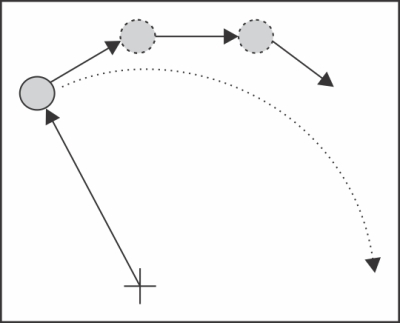In a more schematic way, to calculate the particle position at time dt we have to carry out the following steps:

1. Calculate the direction of motion: `v_x`, `v_y`.

2. Calculate the displacement (`d_x`, `d_y`) which is the product of time and speed and follows the direction of motion.

3. Repeat steps 1 and 2 for enough time steps to cover the total time dt.

The following code shows the full `ParticleSimulator` implementation:

```class ParticleSimulator:

def __init__(self, particles):
self.particles = particles

def evolve(self, dt):
timestep = 0.00001
nsteps = int(dt/timestep)

for i in range(nsteps):
for p in self.particles:

# 1. calculate the direction
norm = (p.x**2 + p.y**2)**0.5
v_x = (-p.y)/norm
v_y = p.x/norm

# 2. calculate the displacement
d_x = timestep * p.ang_speed * v_x
d_y = timestep * p.ang_speed * v_y

p.x += d_x
p.y += d_y
# 3. repeat for all the time steps
```

We can use the `matplotlib` library to visualize our particles. This library is not included in the Python standard library. To install it, you can follow the instructions included in the official documentation at:

### Note

Alternatively, you can use the Anaconda Python distribution (https://store.continuum.io/cshop/anaconda/) that includes `matplotlib` and most of the other third-party packages used in this book. Anaconda is free and available for Linux, Windows, and Mac.

The `plot` function included in `matplotlib` can display our particles as points on a Cartesian grid and the `FuncAnimation` class can animate the evolution of our particles over time.

The `visualize` function accomplishes this by taking the particle simulator and displaying the trajectory in an animated plot.

The `visualize` function is structured as follows:

• Setup the axes and display the particles as points using the plot function

• Write an initialization function (`init`) and an update function (`animate`) that changes the x, y coordinates of the data points using the `line.set_data` method

• Create a `FuncAnimation` instance passing the functions and some parameters

• Run the animation with `plt.show()`

The complete implementation of the visualize function is as follows:

```from matplotlib import pyplot as plt
from matplotlib import animation

def visualize(simulator):

X = [p.x for p in simulator.particles]
Y = [p.y for p in simulator.particles]

fig = plt.figure()
ax = plt.subplot(111, aspect='equal')
line, = ax.plot(X, Y, 'ro')

# Axis limits
plt.xlim(-1, 1)
plt.ylim(-1, 1)

# It will be run when the animation starts
def init():
line.set_data([], [])
return line,

def animate(i):
# We let the particle evolve for 0.1 time units
simulator.evolve(0.01)
X = [p.x for p in simulator.particles]
Y = [p.y for p in simulator.particles]

line.set_data(X, Y)
return line,

# Call the animate function each 10 ms
anim = animation.FuncAnimation(fig, animate, init_func=init, blit=True,# Efficient animation
interval=10)
plt.show()```

Finally, we define a small test functionâ€”`test_visualize`â€”that animates a system of three particles rotating in different directions. Note that the third particle completes a round three times faster than the others:

```def test_visualize():
particles = [Particle( 0.3,  0.5, +1),
Particle( 0.0, -0.5, -1),
Particle(-0.1, -0.4, +3)]

simulator = ParticleSimulator(particles)
visualize(simulator)

if __name__ == '__main__':
test_visualize()```

## Writing tests and benchmarks

Now that we have a working simulator, we can start measuring our performance and tuning-up our code, so that our simulator can handle as many particles as possible. The first step in this process is to write a test and a benchmark.

We need a test that checks whether the results produced by the simulation are correct or not. In the optimization process we will rewrite the code to try different solutions; by doing so we may easily introduce bugs. Maintaining a solid test suite is essential to avoid wasting time on broken code.

Our test will take three particle and let the system evolve for 0.1 time units. We then compare our results, up to a certain precision, with those from a reference implementation:

```def test():
particles = [Particle( 0.3,  0.5, +1),
Particle( 0.0, -0.5, -1),
Particle(-0.1, -0.4, +3)]

simulator = ParticleSimulator(particles)

simulator.evolve(0.1)

p0, p1, p2 = particles

def fequal(a, b):
return abs(a - b) < 1e-5

assert fequal(p0.x, 0.2102698450356825)
assert fequal(p0.y, 0.5438635787296997)

assert fequal(p1.x, -0.0993347660567358)
assert fequal(p1.y, -0.4900342888538049)

assert fequal(p2.x,  0.1913585038252641)
assert fequal(p2.y, -0.3652272210744360)

if __name__ == '__main__':
test()```

We also want to write a benchmark that can measure the performance of our application. This will provide an indication of how much we have improved over the previous implementation.

In our benchmark we instantiate 100 `Particle` objects with random coordinates and angular velocity, and feed them to a `ParticleSimulator` class. We then let the system evolve for 0.1 time units:

```from random import uniform

def benchmark():
particles = [Particle(uniform(-1.0, 1.0),
uniform(-1.0, 1.0),
uniform(-1.0, 1.0))
for i in range(1000)]

simulator = ParticleSimulator(particles)
simulator.evolve(0.1)

if __name__ == '__main__':
benchmark()```

You can easily measure the execution time of any process from the command line by using the Unix `time` command:

```\$ time python simul.py
real    0m1.051s
user    0m1.022s
sys    0m0.028s
```

### Note

The `time` command is not available for Windows, but can be found in the `cygwin` shell that you can download from the official website http://www.cygwin.com/.

By default, `time` shows three metrics:

• `real`: The actual time spent in running the process from start to finish, as if it was measured by a human with a stopwatch

• `user`: The cumulative time spent by all the CPUs during the computation

• `sys`: The cumulative time spent by all the CPUs during system-related tasks such as memory allocation

Notice that sometimes `user` + `sys` might be greater than `real`, as multiple processors may work in parallel.

### Tip

`time` also offers several formatting options; for an overview you can explore its manual (by using the `man time` command). If you want a summary of all the metrics available, you can use the `-v` option.

The Unix `time` command is a good way to benchmark your program. To achieve a more accurate measurement, the benchmark should run long enough (in the order of seconds) so that the setup and tear-down of the process become small, compared to the execution time. The `user` metric is suitable as a monitor for the CPU performance, as the `real` metric includes also the time spent in other processes or waiting for I/O operations.

Another useful program to time Python scripts is the `timeit` module. This module runs a snippet of code in a loop for n times and measures the time taken. Then, it repeats this operation r times (by default the value of r is 3) and takes the best of those runs. Because of this procedure, `timeit` is suitable to accurately time small statements in isolation.

The `timeit` module can be used as a Python module, from the command line, or from IPython.

IPython is a Python shell designed for interactive usage. It boosts tab completion and many utilities to time, profile, and debug your code. We will make use of this shell to try out snippets throughout the book. The IPython shell accepts magic commandsâ€”statements that start with a `%` symbolâ€”that enhance the shell with special behaviors. Commands that start with `%%` are called cell magics, and these commands can be applied on multi-line snippets (called cells).

IPython is available on most Linux distributions and is included in Anaconda. You can follow the installation instructions in the official documentation at:

### Tip

You can use IPython as a regular Python shell (`ipython`) but it is also available in a Qt-based version (`ipython qtconsole`) and as a powerful browser-based interface (`ipython notebook`).

In IPython and command line interfaces it is possible to specify the number of loops or repetitions with the options `-n` and `-r`, otherwise they will be determined automatically. When invoking `timeit` from the command line, you can also give a setup code that will run before executing the statement in a loop.

In the following code we show how to use timeit from IPython, from the command line and as a Python module:

```# IPython Interface
\$ ipython
In : from simul import benchmark
In : %timeit benchmark()
1 loops, best of 3: 782 ms per loop

# Command Line Interface
\$ python -m timeit -s 'from simul import benchmark' 'benchmark()'10 loops, best of 3: 826 msec per loop

# Python Interface
# put this function into the simul.py script

import timeit
result = timeit.timeit('benchmark()',
setup='from __main__ import benchmark', number=10)
# result is the time (in seconds) to run the whole loop

result = timeit.repeat('benchmark()', setup='from __main__ import benchmark', number=10, repeat=3)
# result is a list containing the time of each repetition (repeat=3 in this case)```

Notice that while the command line and IPython interfaces are automatically determining a reasonable value for `n`, the Python interface requires you to explicitly pass it as the `number` argument.

## Finding bottlenecks with cProfile

After assessing the execution time of the program we are ready to identify the parts of the code that need performance tuning. Those parts are typically quite small, compared to the size of the program.

Historically, there are three different profiling modules in Python's standard library:

• The `profile` module: This module is written in pure Python and adds a significant overhead to the program execution. Its presence in the standard library is due mainly to its extendibility.

• The `hotshot` module: A C module designed to minimize the profiling overhead. Its use is not recommended by the Python community and it is not available in Python 3.

• The `cProfile` module: The main profiling module, with an interface similar to `profile`. It has a small overhead and it is suitable as a general purpose profiler.

We will see how to use the cProfile module in two different ways:

• From the command line

• From IPython

In order to use `cProfile`, no change in the code is required, it can be executed directly on an existing Python script or function.

You can use `cProfile` from the command line in this way:

```\$ python -m cProfile simul.py
```

This will print a long output containing several profiling metrics. You can use the option `-s` to sort the output by a certain metric:

```\$ python -m cProfile -s tottime simul.py
```

You can save an output file in a format readable by the `stats` module and other tools by passing the `-o` option:

```\$ python -m cProfile -o prof.out simul.py
```

You can also profile interactively from IPython. The `%prun` magic command lets you profile a function using `cProfile`:

```In : from simul import benchmark
In : %prun benchmark()
707 function calls in 0.793 seconds

Ordered by: internal time

ncalls  tottime  percall  cumtime  percall filename:lineno(function)
1    0.792    0.792    0.792    0.792 simul.py:12(evolve)
1    0.000    0.000    0.000    0.000 simul.py:100(<listcomp>)
300    0.000    0.000    0.000    0.000 random.py:331(uniform)
100    0.000    0.000    0.000    0.000 simul.py:2(__init__)
1    0.000    0.000    0.793    0.793 {built-in method exec}
300    0.000    0.000    0.000    0.000 {method 'random' of '_random.Random' objects}
1    0.000    0.000    0.793    0.793 simul.py:99(benchmark)
1    0.000    0.000    0.793    0.793 <string>:1(<module>)
1    0.000    0.000    0.000    0.000 simul.py:9(__init__)
1    0.000    0.000    0.000    0.000 {method 'disable' of '_lsprof.Profiler' objects}```

The `cProfile` output is divided into five columns:

• `ncalls`: The number of times the function was called.

• `tottime`: The total time spent in the function without taking into account the calls to other functions.

• `cumtime`: The time spent in the function including other function calls.

• `percall`: The time spent for a single call of the functionâ€”it can be obtained by dividing the total or cumulative time by the number of calls.

• `filename:lineno`: The filename and corresponding line number. This information is not present when calling C extensions modules.

The most important metric is `tottime`, the actual time spent in the function body excluding sub-calls. In our case, the largest portion of time is spent in the `evolve` function. We can imagine that the loop is the section of the code that needs performance tuning.

Analyzing data in a textual way can be daunting for big programs with a lot of calls and sub-calls. Some graphic tools aid the task by improving the navigation with an interactive interface.

KCachegrind is a GUI (Graphical User Interface) useful to analyze the profiling output of different programs.

### Note

KCachegrind is available in Ubuntu 13.10 official repositories. The Qt port, QCacheGrind can be downloaded for Windows from the following web page:

Mac users can compile QCacheGrind using Mac Ports (http://www.macports.org/) by following the instructions present in the blog post at this link:

KCachegrind can't read directly the output files produced by `cProfile`. Luckily, the `pyprof2calltree` third-party Python module is able to convert the `cProfile` output file into a format readable by KCachegrind.

You can install `pyprof2calltree` from source (https://pypi.python.org/pypi/pyprof2calltree/) or from the Python Package Index (https://pypi.python.org/).

To best show the KCachegrind features we will use another example with a more diversified structure. We define a recursive function `factorial`, and two other functions that use `factorial`, and they are `taylor_exp` and `taylor_sin`. They represent the polynomial coefficients of the Taylor approximations of `exp(x)` and `sin(x)`:

```def factorial(n):
if n == 0:
return 1.0
else:
return float(n) * factorial(n-1)

def taylor_exp(n):
return [1.0/factorial(i) for i in range(n)]

def taylor_sin(n):
res = []
for i in range(n):
if i % 2 == 1:
res.append((-1)**((i-1)/2)/float(factorial(i)))
else:
res.append(0.0)
return res

def benchmark():
taylor_exp(500)
taylor_sin(500)

if __name__ == '__main__':
benchmark()```

We need to first generate the `cProfile` output file:

```\$ python -m cProfile -o prof.out taylor.py
```

Then, we can convert the output file with `pyprof2calltree` and launch KCachegrind:

```\$ pyprof2calltree -i prof.out -o prof.calltree
\$ kcachegrind prof.calltree # or qcachegrind prof.calltree
```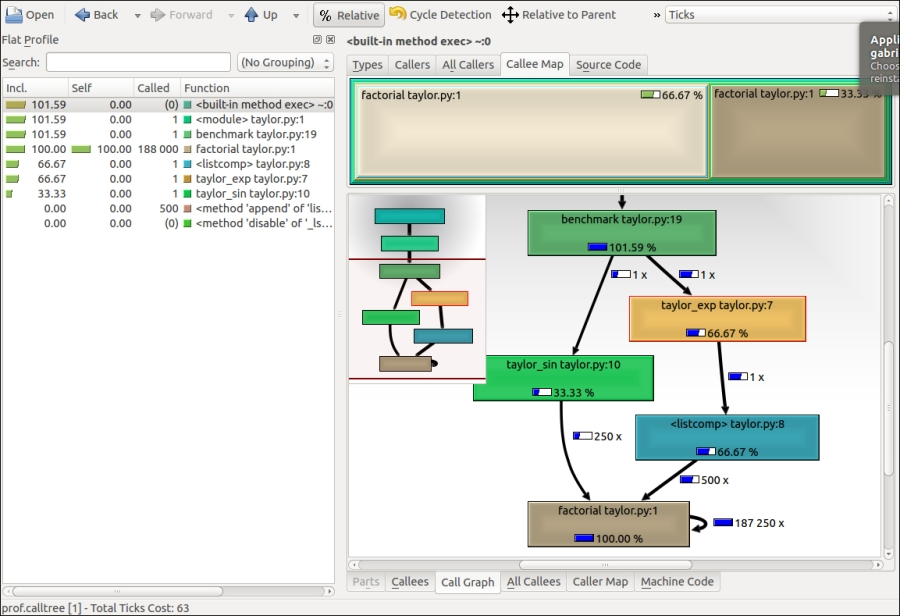The preceding image is a screenshot of the KCachegrind user interface. On the left we have an output fairly similar to `cProfile`. The actual column names are slightly different: Incl. translates to `cProfile` module's `cumtime`; Self translates to `tottime`. The values are given in percentages by clicking on the Relative button on the menu bar. By clicking on the column headers you can sort by the corresponding property.

On the top right, a click on the Callee Map tab contains a diagram of the function costs. In the diagram, each function is represented by a rectangle and the time percentage spent by the function is proportional to the area of the rectangle. Rectangles can contain sub-rectangles that represent sub-calls to other functions. In this case, we can easily see that there are two rectangles for the `factorial` function. The one on the left corresponds to the calls made by `taylor_exp` and the one on the right to the calls made by `taylor_sin`.

On the bottom right, you can display another diagramâ€”the call graphâ€”by clicking on the Call Graph tab. A call graph is a graphical representation of the calling relationship between the functions: each square represents a function and the arrows imply a calling relationship. For example, `taylor_exp` calls <listcomp> (a list comprehension) which calls `factorial` 500 times `taylor_sin` calls factorial 250 times. KCachegrind also detects recursive calls: `factorial` calls itself 187250 times.

You can navigate to the Call Graph or the Caller Map tabs by double-clicking on the rectangles; the interface will update accordingly showing that the timing properties are relative to the selected function. For example, double-clicking on `taylor_exp` will cause the graph to change, showing only the `taylor_exp` contribution to the total cost.

### Note

Gprof2Dot (https://code.google.com/p/jrfonseca/wiki/Gprof2Dot) is another popular tool used to produce call graphs. Starting from output files produced by one of the supported profilers, it will generate a `.dot` diagram representing the call graph.

## Profile line by line with line_profiler

Now that we know which function we have to optimize, we can use the `line_profiler` module that shows us how time is spent in a line-by-line fashion. This is very useful in situations where it's difficult to determine which statements are costly. The `line_profiler` module is a third-party module that is available on the Python Package Index and can be installed by following the instructions on its website:

In order to use `line_profiler`, we need to apply a `@profile` decorator to the functions we intend to monitor. Notice that you don't have to import the `profile` function from another module, as it gets injected in the global namespace when running the profiling script `kernprof.py`. To produce profiling output for our program we need to add the `@profile` decorator to the `evolve` function:

```@profile
def evolve:
# code```

The script `kernprof.py` will produce an output file and will print on standard output the result of the profiling. We should run the script with two options:

• `-l` to use the `line_profiler` function

• `-v` to immediately print the results on screen

```\$ kernprof.py -l -v simul.py
```

It is also possible to run the profiler in an IPython shell for interactive editing. You should first load the `line_profiler` extension that will provide the magic command `lprun`. By using that command you can avoid adding the `@profile` decorator.

```In : %load_ext line_profiler
In : from simul import benchmark, ParticleSimulator
In : %lprun -f ParticleSimulator.evolve benchmark()

Timer unit: 1e-06 s

File: simul.py
Function: evolve at line 12
Total time: 5.31684 s

Line #      Hits         Time  Per Hit   % Time  Line Contents
==============================================================
12                                               def evolve(self, dt):
13         1            9      9.0      0.0          timestep = 0.00001
14         1            4      4.0      0.0          nsteps = int(dt/timestep)
15
16     10001         5837      0.6      0.1          for i in range(nsteps):
17   1010000       517504      0.5      9.7              for p
in self.particles:
18
19   1000000       963498      1.0     18.1                  norm = (p.x**2 + p.y**2)**0.5
20   1000000       621063      0.6     11.7                  v_x = (-p.y)/norm
21   1000000       577882      0.6     10.9                  v_y = p.x/norm
22
23   1000000       672811      0.7     12.7                  d_x = timestep * p.ang_speed * v_x
24   1000000       685092      0.7     12.9                  d_y = timestep * p.ang_speed * v_y
25
26   1000000       650802      0.7     12.2                  p.x += d_x
27   1000000       622337      0.6     11.7                  p.y += d_y```

The output is quite intuitive and is divided into columns:

• Line number: The number of the line that was run

• Hits: The number of times that line was run

• Time: The execution time of the line in microseconds (Time)

• Per Hit: Time divided by hits

• % Time: Fraction of the total time spent executing that line

• Line Contents: the source of the corresponding line

By looking at the percentage column we can have a pretty good idea of where the time is spent. In this case, there are a few statements in the `for` loop body with a cost of around 10-20 percent each.

## Optimizing our code

Now that we have identified exactly how the time is spent, we can modify the code and assess the change in performance.

There are a few different ways to tune up our pure Python code. The way that usually produces the most remarkable results is to change the algorithm. In this case, instead of calculating the velocity and adding small steps, it would be more efficient (and correct, as it is not an approximation) to express the equations of motion in terms of radius `r` and angle `alpha` (instead of `x` and `y`), and then calculate the points on a circle using the equation:

```x = r * cos(alpha)
y = r * sin(alpha)```

Another way lies in minimizing the number of instructions. For example, we can pre-calculate the factor `timestep * p.ang_speed` that doesn't change with time. We can exchange the loop order (first we iterate on particles, then we iterate on time steps) and put the calculation of the factor outside of the loop on the particles.

The line by line profiling showed also that even simple assignment operations can take a considerable amount of time. For example, the following statement takes more than 10 percent of the total time:

` v_x = (-p.y)/norm`

Therefore, a way to optimize the loop is reducing the number of assignment operations. To do that, we can avoid intermediate variables by sacrificing readability and rewriting the expression in a single and slightly more complex statement (notice that the right-hand side gets evaluated completely before being assigned to the variables):

`p.x, p.y = p.x - t_x_ang*p.y/norm, p.y + t_x_ang * p.x/norm`

This leads to the following code:

```    def evolve_fast(self, dt):
timestep = 0.00001
nsteps = int(dt/timestep)

# Loop order is changed
for p in self.particles:
t_x_ang = timestep * p.ang_speed
for i in range(nsteps):
norm = (p.x**2 + p.y**2)**0.5
p.x, p.y = (p.x - t_x_ang *  p.y/norm,p.y + t_x_ang * p.x/norm)
```

After applying the changes we should make sure that the result is still the same, by running our test. We can then compare the execution times using our benchmark:

```\$ time python simul.py # Performance Tuned
real    0m0.756s
user    0m0.714s
sys    0m0.036s

\$ time python simul.py # Original
real    0m0.863s
user    0m0.831s
sys    0m0.028s
```

By acting on pure Python we obtained just a modest increment in speed.

## The dis module

Sometimes, it's not easy to evaluate how many operations a Python statement will take. In this section, we will explore Python internals to estimate the performance of Python statements. Python code gets converted to an intermediate representationâ€”called bytecodeâ€”that gets executed by the Python virtual machine.

To help inspect how the code gets converted into bytecode we can use the Python module `dis` (disassemble). Its usage is really simple, it is sufficient to call the function `dis.dis` on the `ParticleSimulator.evolve` method:

```import dis
from simul import ParticleSimulator
dis.dis(ParticleSimulator.evolve)
```

This will generate, for each line, a list of bytecode instructions. For example, the statement `v_x = (-p.y)/norm` is expanded in the following set of instructions:

```20         85 LOAD_FAST                5 (p)
91 UNARY_NEGATIVE
95 BINARY_TRUE_DIVIDE
96 STORE_FAST               7 (v_x)```

`LOAD_FAST` loads a reference of the variable `p` onto the stack, `LOAD_ATTR` loads the `y` attribute of the item present on top of the stack. The other instructions (`UNARY_NEGATIVE` and `BINARY_TRUE_DIVIDE`) simply do arithmetic operations on top-of-stack items. Finally, the result is stored in `v_x` (`STORE_FAST`).

By analyzing the complete `dis` output we can see that the first version of the loop produces 51 bytecode instructions, while the second gets converted into 35 instructions.

The `dis` module helps discover how the statements get converted and serve mainly as an exploration and learning tool of the Python bytecode representation.

To improve our performance even further, we could keep trying to figure out other approaches to reduce the amount of instructions. It's clear however, that this approach has some limits and it is probably not the right tool for the job. In the next chapter, we will see how to speed up those kinds of calculations with the help of NumPy.

## Profiling memory usage with memory_profiler

In some cases, memory usage constitutes an issue. For example, if we want to handle a huge number of particles we will have a memory overhead due to the creation of many `Particle` instances.

The module `memory_profiler` summarizes, in a way similar to `line_profiler`, the memory usage of the process.

### Note

The `memory_profiler` package is also available on the Python Package Index. You should also install the `psutil` module (https://code.google.com/p/psutil/) as an optional dependency, it will make `memory_profiler` run considerably faster.

Just like `line_profiler`, `memory_profiler` also requires the instrumentation of the source code, by putting a `@profile` decorator on the function we intend to monitor. In our case, we want to analyze the function `benchmark`.

We can slightly change `benchmark` to instantiate a considerable amount (100000) of `Particle` instances and decrease the simulation time:

```def benchmark_memory():
particles = [Particle(uniform(-1.0, 1.0),
uniform(-1.0, 1.0),
uniform(-1.0, 1.0))
for i in range(100000)]

simulator = ParticleSimulator(particles)
simulator.evolve(0.001)```

We can use `memory_profiler` from an IPython shell through the magic command `%mprun`:

```In : %load_ext memory_profiler
In : from simul import benchmark_memory
In : %mprun -f benchmark_memory benchmark_memory()

Line #    Mem usage    Increment   Line Contents
==============================================
135     45.5 MiB      0.0 MiB   def benchmark_memory():
136     45.5 MiB      0.0 MiB       particles = [Particle(uniform(-1.0, 1.0),
137                                                       uniform(-1.0, 1.0),
138                                                       uniform(-1.0, 1.0))
139     71.2 MiB     25.7 MiB                     for i in range(100000)]
140
141     71.2 MiB      0.0 MiB       simulator = ParticleSimulator(particles)
142     71.3 MiB      0.1 MiB       simulator.evolve(0.001)```

### Tip

It is possible to run `memory_profiler` from the shell using the `mprof run` command after adding the `@profile` decorator.

From the output we can see that 100000 `Particle` objects take 25.7 MiB of memory.

### Tip

1 MiB (mebibyte) is equivalent to 10242 = 1,048,576 bytes. It is different from 1 MB (megabyte), which is equivalent to 10002 = 1,000,000 bytes.

We can use `__slots__` on the `Particle` class to reduce its memory footprint. This feature saves some memory by avoiding storing the variables of the instance in an internal dictionary. This optimization has a drawback: it prevents the addition of attributes other than the ones specified in `__slots__ `(to use this feature in Python 2 you should make sure that you are using new-style classes):

```class Particle:
# class Particle(object):  # New-style class for Python 2

__slots__ = ('x', 'y', 'ang_speed')

def __init__(self, x, y, ang_speed):
self.x = x
self.y = y
self.ang_speed = ang_speedWe can now re-run our benchmark:
In : from simul import benchmark_memory
In : %mprun -f benchmark_memory benchmark_memory()

Line #    Mem usage    Increment   Line Contents
==============================================
138     45.5 MiB      0.0 MiB   def benchmark_memory():
139     45.5 MiB      0.0 MiB       particles = [Particle(uniform(-1.0, 1.0),
140                                                       uniform(-1.0, 1.0),
141                                                       uniform(-1.0, 1.0))
142     60.2 MiB     14.7 MiB                     for i in range(100000)]
143
144     60.2 MiB      0.0 MiB       simulator = ParticleSimulator(particles)
145     60.3 MiB      0.1 MiB       simulator.evolve(0.001)```

By rewriting the `Particle` class using `__slots__` we can save 11 MiB of memory.

## Performance tuning tips for pure Python code

As a rule of thumb, when optimizing pure Python code, you should look at what is available in the standard library. The standard library contains clever algorithms for the most common data structures such as lists, dicts, and sets. Furthermore, a lot of standard library modules are implemented in C and have fast processing times. However, it's important to always time the different solutionsâ€”the outcomes are often unpredictable.

The `collections` module provides extra data containers that can efficiently handle some common operations. For example, you can use `deque` in place of a list when you need to pop items from the start and append new items at the end. The `collections` module also includes a `Counter` class that can be used to count repeated elements in an iterable object. Beware, that `Counter` can be slower than the equivalent code written with a standard loop over a dictionary:

```def counter_1():
items = [random.randint(0, 10) for i in range(10000)]
return Counter(items)

def counter_2():
items = [random.randint(0, 10) for i in range(10000)]
counter = {}
for item in items:
if item not in counter:
counter[item] = 0
else:
counter[item] += 1
return counter```

You can put the code in a file named `purepy.py` and time it through IPython:

```In : import purepy
In : %timeit purepy.counter_1()
100 loops, best of 3: 10.1 ms per loop
In : %timeit purepy.counter_2()
100 loops, best of 3: 9.11 ms per loop
```

In general, list comprehension and generators should be preferred in place of explicit loops. Even if the speedup over a standard loop is modest, this is a good practice because it improves readability. We can see in the following example, that both list comprehension and generator expressions are faster than an explicit loop when combined with the function `sum`:

```def loop():
res = []
for i in range(100000):
res.append(i * i)
return sum(res)

def comprehension():
return sum([i * i for i in range(100000)])

def generator():
return sum(i * i for i in range(100000))```

We can add those functions to `purepy.py` and test with IPython:

```In : import purepy
In : %timeit purepy.loop()
100 loops, best of 3: 8.26 ms per loop
In : %timeit purepy.comprehension()
100 loops, best of 3: 5.39 ms per loop
In : %timeit purepy.generator()
100 loops, best of 3: 5.07 ms per loop
```

The `bisect` module can help with fast insertion and retrieval of elements, while maintaining a sorted list.

Raw optimization of pure Python code is not very effective, unless there is a substantial algorithmic advantage. The second-best way to speed up your code is to use external libraries specifically designed for the purpose, such as `numpy`, or to write extensions modules in a more "down to the metal" language such as C with the help of Cython.

## Summary

In this chapter, we introduced the basic principles of optimization and we applied those principles to our test application. The most important thing is identifying the bottlenecks in the application before editing the code. We saw how to write and time a benchmark using the `time` Unix command and the Python `timeit` module. We learned how to profile our application using `cProfile`, `line_profiler`, and `memory_profiler`, and how to analyze and navigate graphically the profiling data with KCachegrind. We surveyed some of the strategies to optimize pure Python code by leveraging the tools available in the standard library.

In the next chapter, we will see how to use `numpy` to dramatically speedup computations in an easy and convenient way.